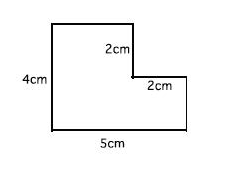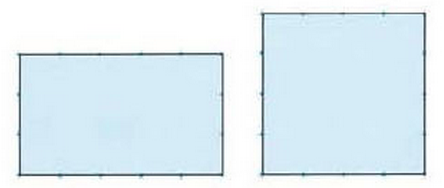# 3.1: Explore the Content

In this module, you will see how an experienced TTP practitioner plans and teaches a series of TTP lessons. Specifically, you will discover how the different instructional supports for a TTP lesson come together to build students’ understanding of a particular mathematical topic: area of polygons.

## 3.1 Examine the Content

To help students build the concepts in the mathematics curriculum– rather than be told those concepts –teachers need to know what their students currently know. Teachers also need to imagine how students might use their current knowledge to build the next mathematics in the curriculum. For these reasons, the first part of TTP lesson planning is investigating the curriculum content and students’ current understanding of it. When you do this in your own setting, you can draw on your own curriculum and standards.

Investigate Standards & Curriculum

Imagine that you want to plan a TTP lesson around the L-shape task below:You first need to understand the unit context and larger mathematical trajectory into which this task fits. What role does this task play in building bigger mathematical ideas? What do students need to know to solve it? What are the important insights students might gain from solving this task?

Your Teacher’s Edition may explain the rationale for each task and how it relates to the unit and content trajectory. Or you may need to consult additional resources. In the case of this task, the Common Core State Standards and related Progressions for Geometry (especially pages 2-5 and p.16) and Geometric Measurement (especially pages 16-18) identify two important understandings that elementary students need to develop.

Composing and decomposing. Composing and decomposing figures provides an opportunity for students to learn about area as the “amount of two-dimensional surface that is contained within a plane figure” and to learn that “area is additive, i.e, the area of the union of two regions that overlap only at their boundaries is the sum of their areas” (CCSS progression geometry measurement K-5, 2012, p.4).

Spatial structuring. To measure area, students must mentally structure the space within a figure–for example, mentally structure a rectangle into rows and columns of square iterated units. The CCSS Progression for Geometry (p.4) notes that “Such spatial structuring precedes meaningful mathematical use of the structures, including multiplication and, later area….” and that “Early composition and decomposition of shape is a foundation for spatial structuring.” Students who are still developing an understanding of area may overlap or gap square units as they attempt to measure.

Below is a lesson plan developed by Dr.Takahashi that includes an explanation of the unit context and larger mathematical trajectory into which this task fits.

Try the Problem and Anticipate Student Thinking

A next crucial step in planning a TTP lesson is to solve the lesson task and anticipate how students will solve it. Solve the tasks below and consider the following questions:

• How will students use what they already know to solve each problem?
• What will be common solution methods? What will be common mistakes and stumbling blocks? Why do these mistakes occur?
• What big mathematical ideas would we like students to learn by solving and discussing this task?

The two polygon area tasks shown below are well-suited to TTP. Depending on what students already understand, one or both tasks might be used within a unit plan. If students do not yet firmly grasp what area is, Problem 1 might be a good way to begin the unit. Once students understand rectangle area and its measurement, Problem 2 provides a good opportunity to see whether students can apply their knowledge to an L-shaped figure. So the first important step in planning a TTP lesson is understanding the unit goals, understanding what students know, and choosing the task that will help students take the crucial next step in their learning.

#### Problem 1

Which of the following shapes, the rectangle or the square, has the larger area, and by how much? Think about a way to express how large they are.Problem What might this problem reveal about student-thinking? What kind of understanding might it build? Compare the area of a square and rectangle (perimeter of 16; area of 16 & 15) Do students grasp the basic concept of area as 2-D space covered? Do students distinguish between area and linear measurement? Do students use direct comparison (overlap) and indirect comparison (using a third object) to compare area? Do students think about units (standard or non-standard) as a way to compare area? Do students accurately structure the space within the square and rectangle into units? Do students accurately use square units to compare area? For example, do they measure without gaps and overlaps of units? Do students grasp the connection between linear measurement and the number of rows, columns and square units? Do students understand why and how to use multiplication to find area?

#### Problem 2

Make the following shape on a geoboard (or dot paper) and find the area. (From “Can You Find the Area?” series of lessons on video by Akihiko Takahashi.)Problem What might this problem reveal about student-thinking? What kind of understanding might it build? Area of L-shape Do students see that the L-shape can be decomposed in various ways? That it can be conceived as part of a larger rectangle? Do they see the additive nature of area? Do they accurately structure the space inside the figure into square units? Do they understand what needs to be done in order to use multiplication to find the area of the L-shape? Do they write mathematical expressions for the total area?